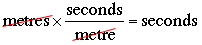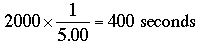SEARCH HOMEMath Central Quandaries & QueriesQuestion from Stephanie, a student: How much time does it take for a student running at an average speed of 5.00 m/s to cover a distance of 2.00 km?Hi Stephanie,

The units are the key here. The problem has the distance in kilometres and the speed in metres per second. I want both length units so I am going to convert the distance of 2 kilometres to 2000 metres since there are 1000 meters in a kilometre.

Now I have a distance in metres, a rate an metres/second and I want the time in seconds.Thus what I want isThis is a lot of seconds so you probably should convert it to minutes and seconds.

PennyMath Central is supported by the University of Regina and The Pacific Institute for the Mathematical Sciences.